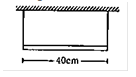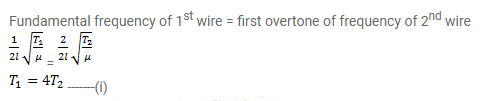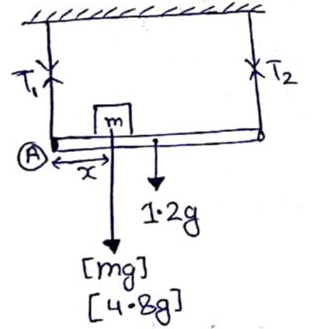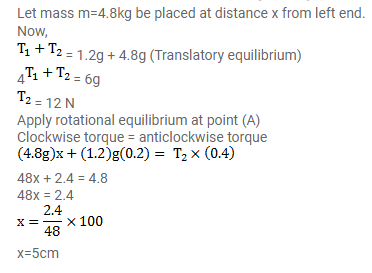# A uniform horizontal rod of length 40 cm and mass 1.2kgQuestion:

A uniform horizontal rod of length $40 \mathrm{~cm}$ and mass $1.2 \mathrm{~kg}$ is supported by two identical wires as shown in figure. Where should a mass of $4.8 \mathrm{~kg}$ be placed on rod so that the same tuning fork may excite the wire on left into its fundamental vibrations and that on right into its first overtone?

Take $\mathrm{g}=10 \mathrm{~m} / \mathrm{s}^{2}$Solution: